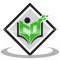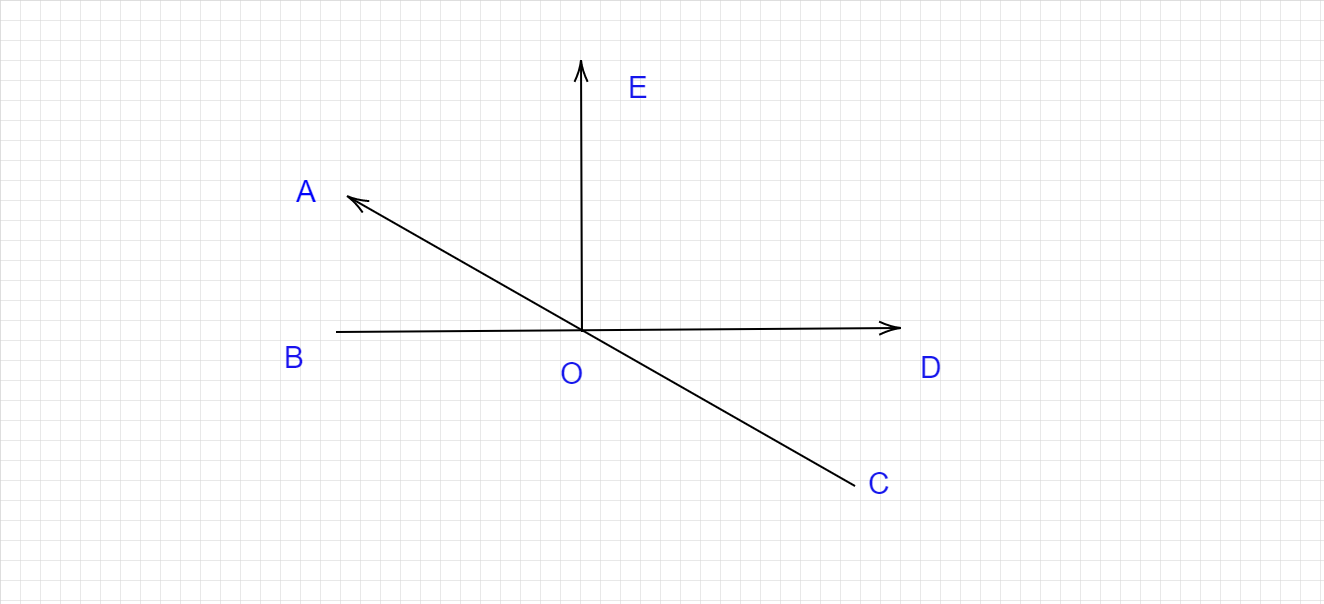## Convert 0.5 into a fraction.Updated on 10-Oct-2022 09:47:26
Given :The given number is 0.5.To do :We have to convert the given decimal into a fraction.Solution :To convert a Decimal to a Fraction follow these stepsStep 1: Write down the decimal divided by 1.Step 2: Multiply both top and bottom by 10 for every number after the decimal point. (For example, if there are two numbers after the decimal point, then use 100, if there are three then use 1000, etc.) Step 3: Simplify (or reduce) the fraction. Given decimal is 0.5.$0.5 = \frac{0.5}{1}$ $\frac{0.5\times 10}{1\times 10} = \frac{5}{10} = \frac{1}{2}$Therefore, 0.5 in fraction form is $\frac{1}{2}$.

## A tank had 500 l of water. A family use one-fifth of the water. How much water was left?Updated on 10-Oct-2022 09:47:26
Given :The volume of water in the tank $= 500 L$The fraction of water used by the family $= \frac{1}{5}$To do :We have to find the water left.Solution :Volume of water used by the family $= \frac{1}{5} (500) L = 100 L$Therefore,Volume of water left in the tank $=$ Total volume $-$ Volume used $= 500 L - 100 L = 400 L$.The water left is 400 L.

## Solve the following:$3 \\frac{2}{5} \\div \\frac{4}{5} of \\frac{1}{5} + \\frac{2}{3} of \\frac{3}{4} - 1 \\frac{35}{72}$.Updated on 10-Oct-2022 09:47:26
Given :The given expression is $3 \frac{2}{5} \div \frac{4}{5} of \frac{1}{5} + \frac{2}{3} of \frac{3}{4} - 1 \frac{35}{72}$.To do:We have to solve the given expression.Solution :We have to use BODMAS in these types of problems.In the Bodmas method, we have to solve in the following order: Brackets first, then of, then division, multiplication, and at last addition and subtraction.$3 \frac{2}{5} \div \frac{4}{5} of \frac{1}{5} + \frac{2}{3} of \frac{3}{4} - 1 \frac{35}{72} = \frac{(3\times 5+2)}{5} \div (\frac{4}{5} \times \frac{1}{5}) + (\frac{2}{3} \times \frac{3}{4}) - \frac{(72\times 1+35)}{72}$                                ... Read More

## Write the difference between weight and mass.Updated on 10-Oct-2022 09:47:26
MASSWEIGHTIt is a measure of the number of atoms or amount of matter in an objectIt is a force exerted by an object of fixed mass due to gravityIt is constant for a body and does not change with placeIt is not constant for a body but it changes from place to placeMeasured using a beam balanceMeasured using a spring balanceSI kgSI N(Newton)

## Write The Factors Of 12,13,14Updated on 10-Oct-2022 09:47:26
Factor: If a number (dividend) is exactly divisible by any number (divisor), then the divisor is a factor of that dividend. Every number has a common factor that is one and the number itself.12 = 1 x 12 = 2 x 6 = 3 x 4 Factors of 12 are 1, 2, 3, 4, 6 and 12.13 = 1 x 13Factors of 13 are 1 and 13.14 = 1 x 14 = 2 x 7Factors of 14 are 1,2,7 and 14.

## In the adjoining figure, name the following pairs of angles.(i) Obtuse vertically opposite angles(ii) Adjacent complementary angles(iii) Equal supplementary angles(iv) Unequal supplementary angles(v) Adjacent angles that do not form a linear"Updated on 10-Oct-2022 09:47:24
Given :The figure of intersecting lines is given.To do :We have to name the given pairs of angles.Solution :(i) Obtuse vertically opposite angles are $∠AOD$ and $∠BOC$.(ii) Adjacent complementary angles are $∠BOA$ and $∠AOE$.(iii) Equal supplementary angles are $∠AOD + ∠DOC = ∠AOB + ∠BOC, ∠BOA + ∠AOD = ∠BOC + ∠COD$ (iv) Unequal supplementary angles are $∠BOE + ∠EOD = ∠BOC + ∠COD, ∠AOE + ∠EOC = ∠AOB + ∠BOC$.(v) Adjacent angles that do not form a linear pair are $∠AOE$ and $∠EOD$; $∠EOD$ and $∠DOC$; $∠DOC$ and $COB; ∠COB$ and $∠BOA; ∠BOE$ and $∠EOD$.

## Shalina got $\\frac{4}{5}$ of total marks in a test. If the total marks were 50, How much did she score?Updated on 10-Oct-2022 09:47:24
Given :Shalina got  $\frac{4}{5}$ of total marks in a test.The total marks of the test $= 50$.To do :We have to find the score.Solution :Marks scored by Shalina $= \frac{4}{5} (50) = 4\times 10 = 40$Therefore, Shalina scored 40 marks in the test.

## What is the difference between distillation and fractional distillation?Updated on 10-Oct-2022 09:47:24
The differences between simple distillation and fractional distillation are as follows:- Simple Distillation Fractional Distillation 1. It is used to separate a mixture of miscible liquids with a sufficiently large difference in their boiling point. 1. It is used when the difference in the boiling point is quite less.2. It consists of a simple apparatus with a condenser and two flasks. 2. It consists of a complex apparatus with a fractionating column. 3. Example: Purify seawater 3. Example: crude oil refining

## Antonie van Leeuwenhoek discovered _____ and ______ in the 16th century.Updated on 31-Mar-2023 12:04:07
Antonie van Leeuwenhoek discovered simple microscope  and blood cells in the 16th century.\r

## If ∠ B and ∠ Q are acute angles such that $Sin B = Sin Q$, then prove that $∠ B = ∠ Q$.Updated on 10-Oct-2022 09:47:24
Given:$Sin B = Sin Q$To do :We have to prove that  $∠ B = ∠ Q$.Solution :Consider two right triangles ABC and PQR where $sin B = sin Q$. From the figures, $Sin B = \frac{AC}{AB}$and $Sin Q = \frac{PR}{PQ}$This implies, $\frac{AC}{AB} = \frac{PR}{PQ}$$\frac{AC}{PR} = \frac{AB}{PQ}Let\frac{AC}{PR} = \frac{AB}{PQ} = k$$AC = k(PR)$ and $AB = k(PQ)$ In triangles, ABC and PQR, using the Pythagoras theorem, $AC^2 + BC^2 = AB^2$ and $QR^2+PR^2=PQ^2$ $BC = \sqrt{(AB^2-AC^2)}$ and $QR = \sqrt{(PQ^2-PR^2)}$ $\frac{BC}{QR} = \frac{ \sqrt{(AB^2-AC^2)}}{\sqrt{(PQ^2-PR^2)}}$$\frac{BC}{QR} = \frac{ \sqrt{(kPQ)^2-(kPR^2)}}{\sqrt{(PQ^2-PR^2)}}$$\frac{BC}{QR} = \frac{ \sqrt{k^2 (PQ^2-PR^2)}}{\sqrt{(PQ^2-PR^2)}}$ $\frac{BC}{QR} = \frac{k \sqrt{(PQ^2-PR^2)}}{\sqrt{(PQ^2-PR^2)}}$$\frac{BC}{QR} = k$ \$\frac{AC}{PR} = \frac{AB}{PQ} = ... Read More